# Composition Stoichiometry Chapter 7 Percent Composition by Mass

• Slides: 12Composition Stoichiometry Chapter 7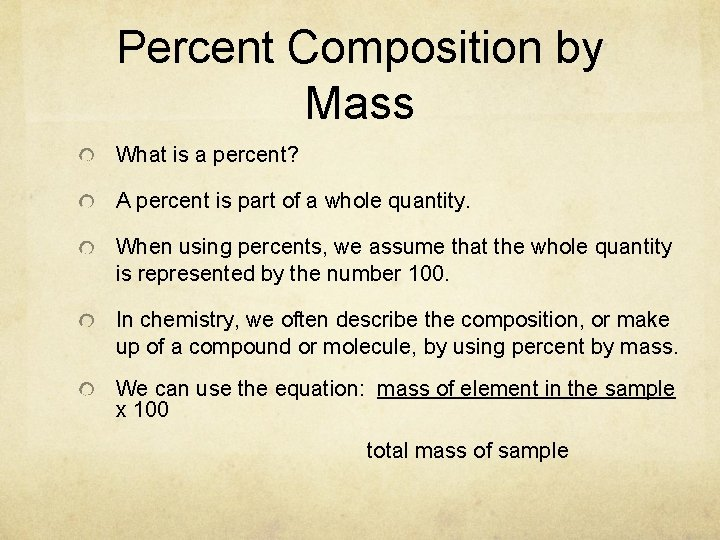Percent Composition by Mass What is a percent? A percent is part of a whole quantity. When using percents, we assume that the whole quantity is represented by the number 100. In chemistry, we often describe the composition, or make up of a compound or molecule, by using percent by mass. We can use the equation: mass of element in the sample x 100 total mass of samplePercent Composition Another way to find percent composition by mass is to use the following equation: mass of element in 1 mole of a compound element molar mass of compound x 100= % of in o Let’s do an example. o Find the percent of each element by mass in copper (I) sulfide, Cu 2 S.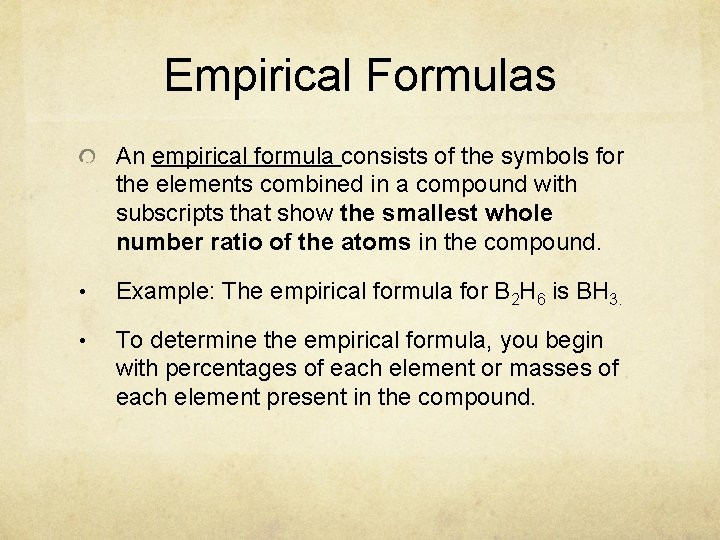Empirical Formulas An empirical formula consists of the symbols for the elements combined in a compound with subscripts that show the smallest whole number ratio of the atoms in the compound. • Example: The empirical formula for B 2 H 6 is BH 3. • To determine the empirical formula, you begin with percentages of each element or masses of each element present in the compound.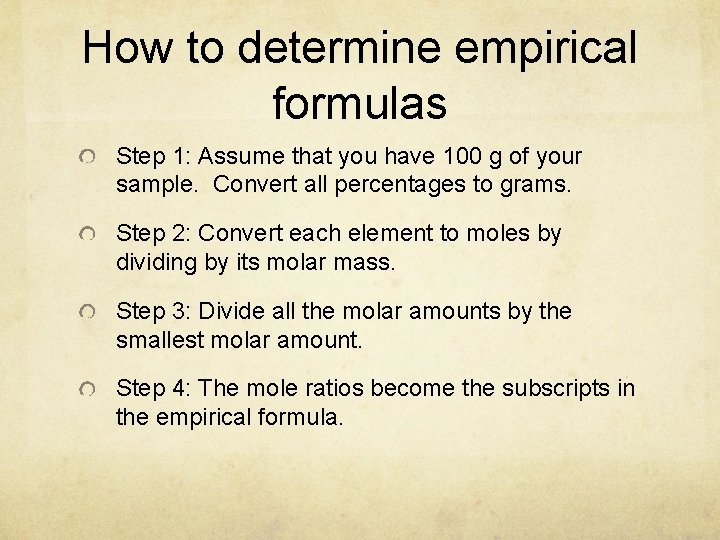How to determine empirical formulas Step 1: Assume that you have 100 g of your sample. Convert all percentages to grams. Step 2: Convert each element to moles by dividing by its molar mass. Step 3: Divide all the molar amounts by the smallest molar amount. Step 4: The mole ratios become the subscripts in the empirical formula.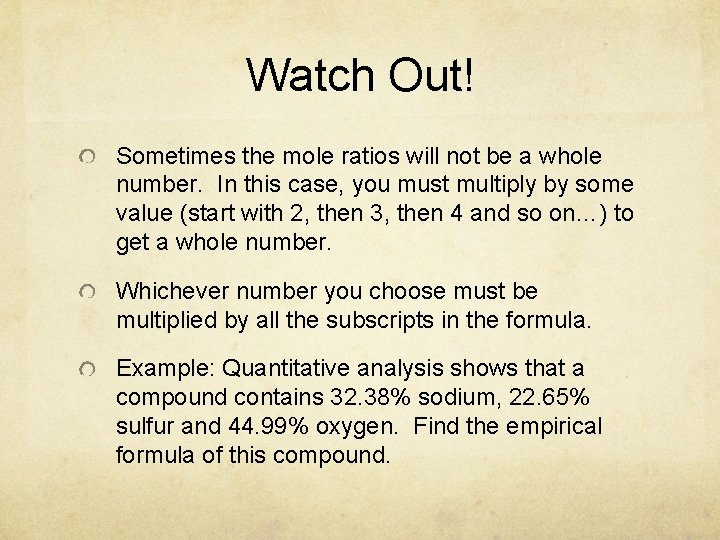Watch Out! Sometimes the mole ratios will not be a whole number. In this case, you must multiply by some value (start with 2, then 3, then 4 and so on…) to get a whole number. Whichever number you choose must be multiplied by all the subscripts in the formula. Example: Quantitative analysis shows that a compound contains 32. 38% sodium, 22. 65% sulfur and 44. 99% oxygen. Find the empirical formula of this compound.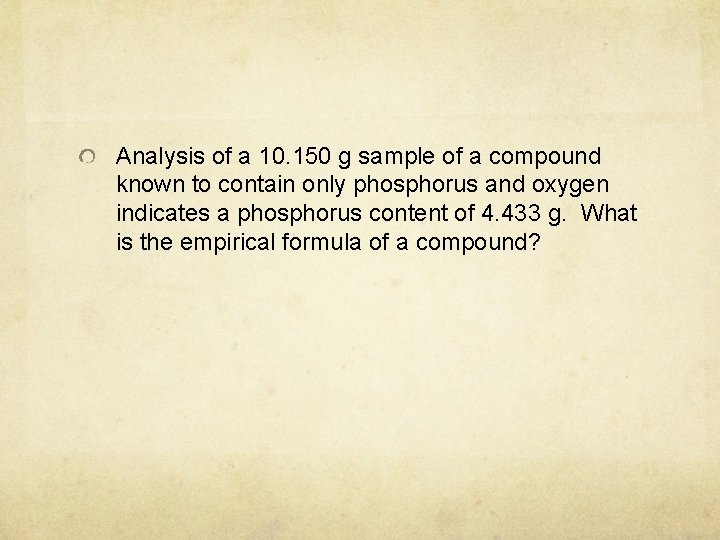Analysis of a 10. 150 g sample of a compound known to contain only phosphorus and oxygen indicates a phosphorus content of 4. 433 g. What is the empirical formula of a compound?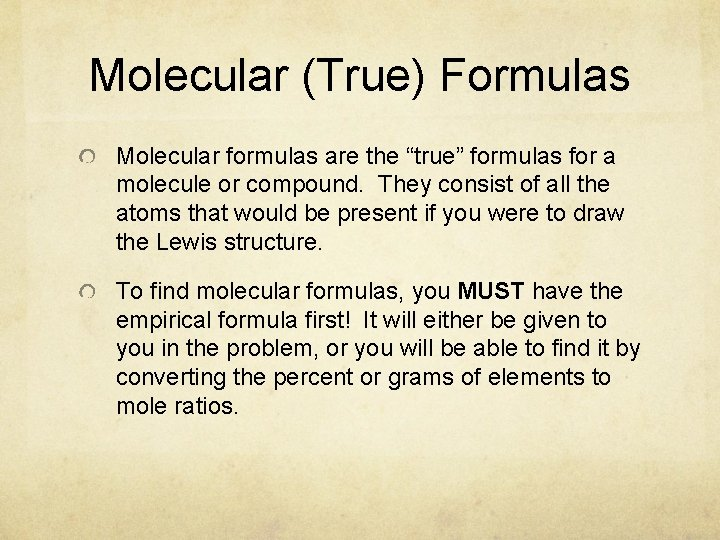Molecular (True) Formulas Molecular formulas are the “true” formulas for a molecule or compound. They consist of all the atoms that would be present if you were to draw the Lewis structure. To find molecular formulas, you MUST have the empirical formula first! It will either be given to you in the problem, or you will be able to find it by converting the percent or grams of elements to mole ratios.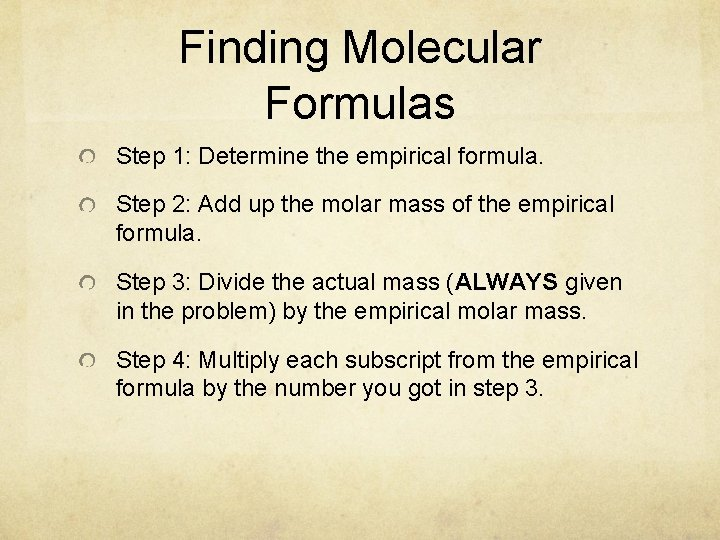Finding Molecular Formulas Step 1: Determine the empirical formula. Step 2: Add up the molar mass of the empirical formula. Step 3: Divide the actual mass (ALWAYS given in the problem) by the empirical molar mass. Step 4: Multiply each subscript from the empirical formula by the number you got in step 3.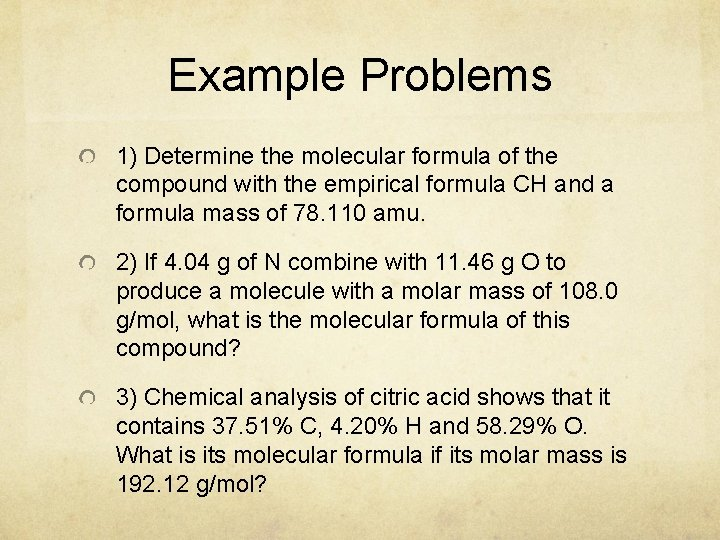Example Problems 1) Determine the molecular formula of the compound with the empirical formula CH and a formula mass of 78. 110 amu. 2) If 4. 04 g of N combine with 11. 46 g O to produce a molecule with a molar mass of 108. 0 g/mol, what is the molecular formula of this compound? 3) Chemical analysis of citric acid shows that it contains 37. 51% C, 4. 20% H and 58. 29% O. What is its molecular formula if its molar mass is 192. 12 g/mol?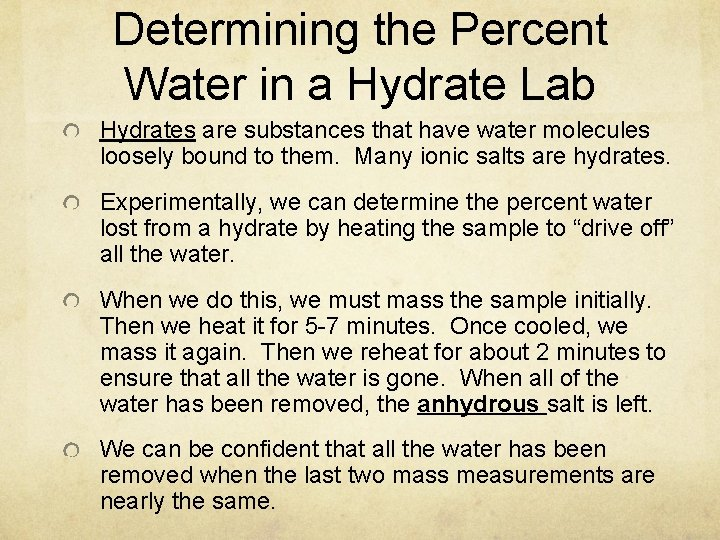Determining the Percent Water in a Hydrate Lab Hydrates are substances that have water molecules loosely bound to them. Many ionic salts are hydrates. Experimentally, we can determine the percent water lost from a hydrate by heating the sample to “drive off” all the water. When we do this, we must mass the sample initially. Then we heat it for 5 -7 minutes. Once cooled, we mass it again. Then we reheat for about 2 minutes to ensure that all the water is gone. When all of the water has been removed, the anhydrous salt is left. We can be confident that all the water has been removed when the last two mass measurements are nearly the same.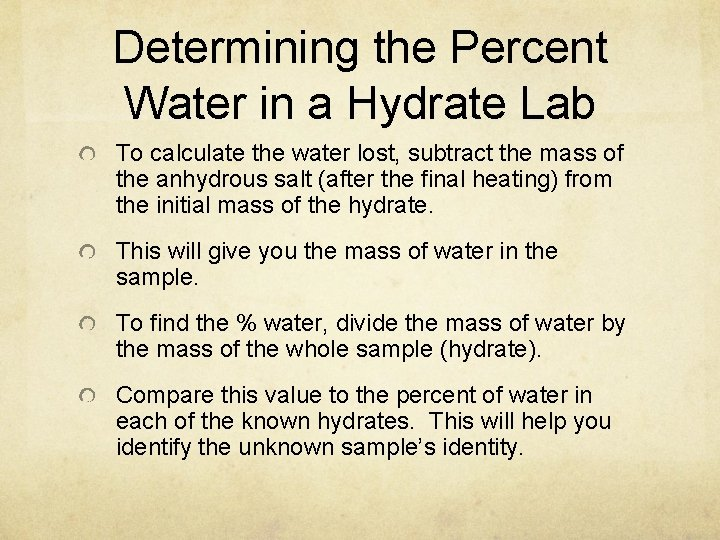Determining the Percent Water in a Hydrate Lab To calculate the water lost, subtract the mass of the anhydrous salt (after the final heating) from the initial mass of the hydrate. This will give you the mass of water in the sample. To find the % water, divide the mass of water by the mass of the whole sample (hydrate). Compare this value to the percent of water in each of the known hydrates. This will help you identify the unknown sample’s identity.